# Chapter 10: Basic Shapes

## 10.1. Introduction and definitions

basic shape
shape
shape elements
A graphics element that is defined by some combination of straight lines and curves. Specifically: circle, ellipse, line, path, polygon, polyline and rect.

SVG contains the following set of basic shape elements:

• rectangles (including optional rounded corners), created with the rect element,
• circles, created with the circle element,
• ellipses, created with the ellipse element,
• straight lines, created with the line element,
• polylines, created with the polyline element, and
• polygons, created with the polygon element

Mathematically, these shape elements are equivalent to a path element that would construct the same shape. The basic shapes may be stroked, filled and used as clip paths. All of the properties available for path elements also apply to the basic shapes.

The equivalent path and algorithm to compute the stroke for each shape are defined in the shape sections below.

## 10.2. The ‘rect’ element

The rect element defines a rectangle which is axis-aligned with the current user coordinate system. Rounded rectangles can be achieved by setting non-zero values for the rx and ry geometric properties.

rect
Categories:
Graphics element, renderable element, shape element
Content model:
Any number of the following elements, in any order:
Attributes:
Geometry properties:
DOM Interfaces:

The x and y coordinates refer to the left and top edges of the rectangle, in the current user coordinate system.

The width and height properties define the overall width and height of the rectangle. A negative value for either property is invalid and must be ignored. A computed value of zero for either dimension disables rendering of the element.

For rounded rectangles, the computed values of the rx and ry properties define the x- and y-axis radii of elliptical arcs used to round off the corners of the rectangle. The arc are always symmetrical along both horizontal and vertical axis; to create a rectangle with uneven corner rounding, define the shape explicitly with a path. A negative value for either property is invalid and must be ignored. A computed value of zero for either dimension, or a computed value of `auto` for both dimensions, results in a rectangle without corner rounding.

The used values for the x- and y-axis rounded corner radii may be determined implicitly from the other dimension (using the `auto` value), and are also subject to clamping so that the lengths of the straight segments of the rectangle are never negative. The used values for rx and ry are determined from the computed values by following these steps in order:

1. If both rx and ry have a computed value of `auto` (since `auto` is the initial value for both properties, this will also occur if neither are specified by the author or if all author-supplied values are invalid), then the used value of both rx and ry is 0. (This will result in square corners.)
2. Otherwise, convert specified values to absolute values as follows:
1. If rx is set to a length value or a percentage, but ry is `auto`, calculate an absolute length equivalent for rx, resolving percentages against the used width of the rectangle; the absolute value for ry is the same.
2. If ry is set to a length value or a percentage, but rx is `auto`, calculate the absolute length equivalent for ry, resolving percentages against the used height of the rectangle; the absolute value for rx is the same.
3. If both rx and ry were set to lengths or percentages, absolute values are generated individually, resolving rx percentages against the used width, and resolving ry percentages against the used height.
3. Finally, apply clamping to generate the used values:
1. If the absolute rx (after the above steps) is greater than half of the used width, then the used value of rx is half of the used width.
2. If the absolute ry (after the above steps) is greater than half of the used height, then the used value of ry is half of the used height.
3. Otherwise, the used values of rx and ry are the absolute values computed previously.

Mathematically, a rect element is mapped to an equivalent path element as follows, after generating absolute used values x, y, width, height, rx, and rx in user units for the user coordinate system, for each of the equivalent geometric properties following the rules specified above and in Units:

1. perform an absolute moveto operation to location (x+rx,y);
2. perform an absolute horizontal lineto with parameter x+width-rx;
3. if both rx and ry are greater than zero, perform an absolute elliptical arc operation to coordinate (x+width,y+ry), where rx and ry are used as the equivalent parameters to the elliptical arc command, the x-axis-rotation and large-arc-flag are set to zero, the sweep-flag is set to one;
4. perform an absolute vertical lineto parameter y+height-ry;
5. if both rx and ry are greater than zero, perform an absolute elliptical arc operation to coordinate (x+width-rx,y+height), using the same parameters as previously;
6. perform an absolute horizontal lineto parameter x+rx;
7. if both rx and ry are greater than zero, perform an absolute elliptical arc operation to coordinate (x,y+height-ry), using the same parameters as previously;
8. perform an absolute vertical lineto parameter y+ry
9. if both rx and ry are greater than zero, perform an absolute elliptical arc operation with a segment-completing close path operation, using the same parameters as previously.

Path decomposition resolved during teleconference on June 3rd, 2013.

Example rect01 shows a rectangle with sharp corners. The rect element is filled with yellow and stroked with navy.

```<?xml version="1.0" standalone="no"?>
<svg width="12cm" height="4cm" viewBox="0 0 1200 400"
xmlns="http://www.w3.org/2000/svg" version="1.1">
<desc>Example rect01 - rectangle with sharp corners</desc>

<!-- Show outline of viewport using 'rect' element -->
<rect x="1" y="1" width="1198" height="398"
fill="none" stroke="blue" stroke-width="2"/>

<rect x="400" y="100" width="400" height="200"
fill="yellow" stroke="navy" stroke-width="10"  />
</svg>```Example rect01

View this example as SVG (SVG-enabled browsers only)

Example rect02 shows two rounded rectangles. The rx specifies how to round the corners of the rectangles. Note that since no value has been specified for the ry attribute, the used value will be derived from the rx attribute.

```<?xml version="1.0" standalone="no"?>
<svg width="12cm" height="4cm" viewBox="0 0 1200 400"
xmlns="http://www.w3.org/2000/svg" version="1.1">
<desc>Example rect02 - rounded rectangles</desc>

<!-- Show outline of viewport using 'rect' element -->
<rect x="1" y="1" width="1198" height="398"
fill="none" stroke="blue" stroke-width="2"/>

<rect x="100" y="100" width="400" height="200" rx="50"
fill="green" />

<g transform="translate(700 210) rotate(-30)">
<rect x="0" y="0" width="400" height="200" rx="50"
fill="none" stroke="purple" stroke-width="30" />
</g>
</svg>```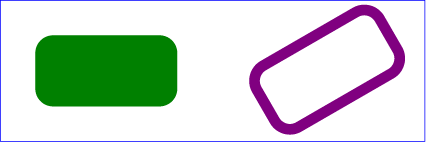Example rect02

View this example as SVG (SVG-enabled browsers only)

## 10.3. The ‘circle’ element

The circle element defines a circle based on a center point and a radius.

circle
Categories:
Graphics element, renderable element, shape element
Content model:
Any number of the following elements, in any order:
Attributes:
Geometry properties:
DOM Interfaces:

The cx and cy attributes define the coordinates of the center of the circle.

The r attribute defines the radius of the circle. A negative value is invalid and must be ignored. A computed value of zero disables rendering of the element.

Mathematically, a circle element is mapped to an equivalent path element that consists of four elliptical arc segments, each covering a quarter of the circle. The path begins at the "3 o'clock" point on the radius and proceeds in a clock-wise direction (before any transformations). The rx and ry parameters to the arc commands are both equal to the used value of the r property, after conversion to local user units, while the x-axis-rotation, the large-arc-flag, and the sweep-flag are all set to zero. The coordinates are computed as follows, where cx, cy, and r are the used values of the equivalent properties, converted to user units:

1. A move-to command to the point cx+r,cy;
2. arc to cx,cy+r;
3. arc to cx-r,cy;
4. arc to cx,cy-r;
5. arc with a segment-completing close path operation.

Path decomposition resolved during teleconference on June 3rd, 2013.

Example circle01 consists of a circle element that is filled with red and stroked with blue.

```<?xml version="1.0" standalone="no"?>
<svg width="12cm" height="4cm" viewBox="0 0 1200 400"
xmlns="http://www.w3.org/2000/svg" version="1.1">
<desc>Example circle01 - circle filled with red and stroked with blue</desc>

<!-- Show outline of viewport using 'rect' element -->
<rect x="1" y="1" width="1198" height="398"
fill="none" stroke="blue" stroke-width="2"/>

<circle cx="600" cy="200" r="100"
fill="red" stroke="blue" stroke-width="10"  />
</svg>```Example circle01

View this example as SVG (SVG-enabled browsers only)

## 10.4. The ‘ellipse’ element

The ellipse element defines an ellipse which is axis-aligned with the current user coordinate system based on a center point and two radii.

ellipse
Categories:
Graphics element, renderable element, shape element
Content model:
Any number of the following elements, in any order:
Attributes:
Geometry properties:
DOM Interfaces:

The cx and cy coordinates define the center of the ellipse.

The rx and ry properties define the x- and y-axis radii of the ellipse. A negative value for either property is invalid and must be ignored. A computed value of zero for either dimension, or a computed value of `auto` for both dimensions, disables rendering of the element.

An `auto` value for either rx or ry is converted to a used value, following the rules given above for rectangles (but without any clamping based on width or height). Effectively, an `auto` value creates a circular shape whose radius is defined by a value expressed solely in one dimension; this allows for creating a circle with a radius defined in terms of one of the following:

• a percentage of the coordinate system width; that is, a percentage value for rx and an `auto` value for ry.
• a percentage of the coordinate system height; that is, an `auto` value for rx and a percentage value for ry.

New in SVG 2. The `auto` value for rx and ry was added to allow consistent parsing of these properties for both ellipses and rectangles. Previously, if either rx or ry was unspecified, the ellipse would not render.

Mathematically, an ellipse element is mapped to an equivalent path element that consists of four elliptical arc segments, each covering a quarter of the ellipse. The path begins at the "3 o'clock" point on the radius and proceeds in a clock-wise direction (before any transformation). The rx and ry parameters to the arc commands are the used values of the equivalent properties after conversion to local user units, while the x-axis-rotation, the large-arc-flag, and the sweep-flag are all set to zero. The coordinates are computed as follows, where cx, cy, rx, and ry are the used values of the equivalent properties, converted to user units:

1. A move-to command to the point cx+rx,cy;
2. arc to cx,cy+ry;
3. arc to cx-rx,cy;
4. arc to cx,cy-ry;
5. arc with a segment-completing close path operation.

Path decomposition resolved during teleconference on June 3rd, 2013.

Example ellipse01 below specifies the coordinates of the two ellipses in the user coordinate system established by the viewBox attribute on the svg element and the transform property on the g and ellipse elements. Both ellipses use the default values of zero for the cx and cy attributes (the center of the ellipse). The second ellipse is rotated.

```<?xml version="1.0" standalone="no"?>
<svg width="12cm" height="4cm" viewBox="0 0 1200 400"
xmlns="http://www.w3.org/2000/svg" version="1.1">
<desc>Example ellipse01 - examples of ellipses</desc>

<!-- Show outline of viewport using 'rect' element -->
<rect x="1" y="1" width="1198" height="398"
fill="none" stroke="blue" stroke-width="2" />

<g transform="translate(300 200)">
<ellipse rx="250" ry="100"
fill="red"  />
</g>

<ellipse transform="translate(900 200) rotate(-30)"
rx="250" ry="100"
fill="none" stroke="blue" stroke-width="20"  />

</svg>```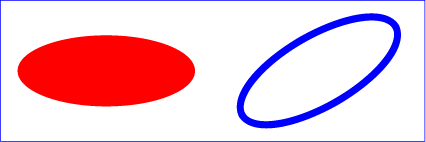Example ellipse01

View this example as SVG (SVG-enabled browsers only)

## 10.5. The ‘line’ element

The line element defines a line segment that starts at one point and ends at another.

line
Categories:
Graphics element, renderable element, shape element
Content model:
Any number of the following elements, in any order:
Attributes:
DOM Interfaces:

Attribute definitions:

Name Value Initial value Animatable
x1, y1 <length-percentage> | <number> 0 yes
The x- and y-axis coordinates of the start of the line.
Name Value Initial value Animatable
x2, y2 <length-percentage> | <number> 0 yes
The x- and y-axis coordinates of the end of the line.

A future specification may convert the x1, y1, x2, and y2 attributes to geometric properties. Currently, they can only be specified via element attributes, and not CSS.

Mathematically, a line element can be mapped to an equivalent path element as follows, after converting coordinates into user coordinate system user units according to Units to generate values x1, y1, x2, and y2:

• perform an absolute moveto operation to absolute location (x1,y1)
• perform an absolute lineto operation to absolute location (x2,y2)

Because line elements are single lines and thus are geometrically one-dimensional, they have no interior; thus, line elements are never filled (see the fill property).

Example line01 below specifies the coordinates of the five lines in the user coordinate system established by the viewBox attribute on the svg element. The lines have different thicknesses.

```<?xml version="1.0" standalone="no"?>
<svg width="12cm" height="4cm" viewBox="0 0 1200 400"
xmlns="http://www.w3.org/2000/svg" version="1.1">
<desc>Example line01 - lines expressed in user coordinates</desc>

<!-- Show outline of viewport using 'rect' element -->
<rect x="1" y="1" width="1198" height="398"
fill="none" stroke="blue" stroke-width="2" />

<g stroke="green" >
<line x1="100" y1="300" x2="300" y2="100"
stroke-width="5"  />
<line x1="300" y1="300" x2="500" y2="100"
stroke-width="10"  />
<line x1="500" y1="300" x2="700" y2="100"
stroke-width="15"  />
<line x1="700" y1="300" x2="900" y2="100"
stroke-width="20"  />
<line x1="900" y1="300" x2="1100" y2="100"
stroke-width="25"  />
</g>
</svg>```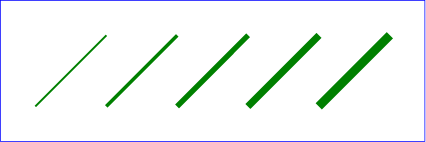Example line01

View this example as SVG (SVG-enabled browsers only)

## 10.6. The ‘polyline’ element

The polyline element defines a set of connected straight line segments. Typically, polyline elements define open shapes.

polyline
Categories:
Graphics element, renderable element, shape element
Content model:
Any number of the following elements, in any order:
Attributes:
DOM Interfaces:

Attribute definitions:

Name Value Initial value Animatable
points <points> (none) yes

where:

<points> =
[ <number>+ ]#

The points that make up the polyline. All coordinate values are in the user coordinate system.

If an odd number of coordinates is provided, then the element is in error, with the same user agent behavior as occurs with an incorrectly specified path element. In such error cases the user agent will drop the last, odd coordinate and otherwise render the shape.

The initial value, (none), indicates that the polyline element is valid but does not render.

A future specification may convert the points attribute to a geometric property. Currently, it can only be specified via an element attribute, and not CSS.

Mathematically, a polyline element can be mapped to an equivalent path element as follows:

• perform an absolute moveto operation to the first coordinate pair in the list of points
• for each subsequent coordinate pair, perform an absolute lineto operation to that coordinate pair.

Example polyline01 below specifies a polyline in the user coordinate system established by the viewBox attribute on the svg element.

```<?xml version="1.0" standalone="no"?>
<svg width="12cm" height="4cm" viewBox="0 0 1200 400"
xmlns="http://www.w3.org/2000/svg" version="1.1">
<desc>Example polyline01 - increasingly larger bars</desc>

<!-- Show outline of viewport using 'rect' element -->
<rect x="1" y="1" width="1198" height="398"
fill="none" stroke="blue" stroke-width="2" />

<polyline fill="none" stroke="blue" stroke-width="10"
points="50,375
150,375 150,325 250,325 250,375
350,375 350,250 450,250 450,375
550,375 550,175 650,175 650,375
750,375 750,100 850,100 850,375
950,375 950,25 1050,25 1050,375
1150,375" />
</svg>```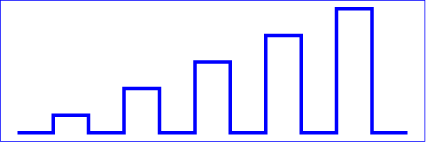Example polyline01

View this example as SVG (SVG-enabled browsers only)

## 10.7. The ‘polygon’ element

The polygon element defines a closed shape consisting of a set of connected straight line segments.

polygon
Categories:
Graphics element, renderable element, shape element
Content model:
Any number of the following elements, in any order:
Attributes:
DOM Interfaces:

Attribute definitions:

Name Value Initial value Animatable
points <points> (none) yes

The points that make up the polygon. All coordinate values are in the user coordinate system.

If an odd number of coordinates is provided, then the element is in error, with the same user agent behavior as occurs with an incorrectly specified path element.

The initial value, (none), indicates that the polygon element is valid, but does not render.

A future specification may convert the points attribute to a geometric property. Currently, it can only be specified via an element attribute, and not CSS.

Mathematically, a polygon element can be mapped to an equivalent path element as follows:

• perform an absolute moveto operation to the first coordinate pair in the list of points
• for each subsequent coordinate pair, perform an absolute lineto operation to that coordinate pair
• perform a closepath command

Example polygon01 below specifies two polygons (a star and a hexagon) in the user coordinate system established by the viewBox attribute on the svg element.

```<?xml version="1.0" standalone="no"?>
<svg width="12cm" height="4cm" viewBox="0 0 1200 400"
xmlns="http://www.w3.org/2000/svg" version="1.1">
<desc>Example polygon01 - star and hexagon</desc>

<!-- Show outline of viewport using 'rect' element -->
<rect x="1" y="1" width="1198" height="398"
fill="none" stroke="blue" stroke-width="2" />

<polygon fill="red" stroke="blue" stroke-width="10"
points="350,75  379,161 469,161 397,215
423,301 350,250 277,301 303,215
231,161 321,161" />
<polygon fill="lime" stroke="blue" stroke-width="10"
points="850,75  958,137.5 958,262.5
850,325 742,262.6 742,137.5" />
</svg>```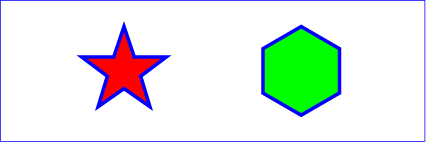Example polygon01

View this example as SVG (SVG-enabled browsers only)

## 10.8. DOM interfaces

### 10.8.1. Interface SVGRectElement

An SVGRectElement object represents a rect element in the DOM.

```[Exposed=Window]
interface SVGRectElement : SVGGeometryElement {
};```

The x, y, width, height, rx and ry IDL attributes reflect the computed values of the x, y, width, height, rx and ry properties and their corresponding presentation attributes, respectively.

### 10.8.2. Interface SVGCircleElement

An SVGCircleElement object represents a circle element in the DOM.

```[Exposed=Window]
interface SVGCircleElement : SVGGeometryElement {
};```

The cx, cy and r IDL attributes reflect the computed values of the cx, cy and y properties and their corresponding presentation attributes, respectively.

### 10.8.3. Interface SVGEllipseElement

An SVGEllipseElement object represents a ellipse element in the DOM.

```[Exposed=Window]
interface SVGEllipseElement : SVGGeometryElement {
};```

The cx, cy, rx and ry IDL attributes reflect the computed values of the cx, cy, rx and ry properties and their corresponding presentation attributes, respectively.

### 10.8.4. Interface SVGLineElement

The SVGLineElement interface corresponds to the line element.
```[Exposed=Window]
interface SVGLineElement : SVGGeometryElement {
};```

The x1, y1, x2 and y2 IDL attributes reflect the x1, y1, x2 and y2 content attributes, respectively

### 10.8.5. Mixin SVGAnimatedPoints

The SVGAnimatedPoints interface is used to reflect a points attribute on a polygon or polyline element. It is mixed in to the SVGPolygonElement and SVGPolylineElement interfaces.

Note: In SVG 1.1 SE, the animatedPoints attribute represented the current animated value. In this version of SVG, it is simply an alias for points.

```interface mixin SVGAnimatedPoints {
};```

The points and animatedPoints IDL attributes represent the current non-animated value of the reflected attribute. On getting points or animatedPoints, an SVGPointList object is returned that reflects the base value of the reflected attribute.

### 10.8.6. Interface SVGPointList

The SVGPointList interface is a list interface whose elements are DOMPoint objects. An SVGPointList object represents a list of points.

```[Exposed=Window]
interface SVGPointList {

undefined clear();
DOMPoint initialize(DOMPoint newItem);
getter DOMPoint getItem(unsigned long index);
DOMPoint insertItemBefore(DOMPoint newItem, unsigned long index);
DOMPoint replaceItem(DOMPoint newItem, unsigned long index);
DOMPoint removeItem(unsigned long index);
DOMPoint appendItem(DOMPoint newItem);
setter undefined (unsigned long index, DOMPoint newItem);
};```

The behavior of all of the interface members of SVGPointList are defined in List interfaces.

This specification imposes additional requirements on the behaviour of DOMPoint objects beyond those described in the Geometry Interfaces specification, so that they can be used to reflect points attributes.

Every DOMPoint object operates in one of three modes. It can:

1. reflect an element of the base value of a reflected animatable attribute (being exposed through the methods on the points member of an SVGAnimatedPoints),
2. represent the current translation of a given svg element (being exposed through the currentTranslate member on SVGSVGElement), or
3. be detached, which is the case for DOMPoint objects created using their constructor or with createSVGPoint.

A DOMPoint object can be associated with a particular element. The associated element is used to determine which element's content attribute to update if the object reflects an attribute. Unless otherwise described, a DOMPoint object is not associated with any element.

A DOMPoint object can be designated as read only, which means that attempts to modify the object will result in an exception being thrown. When assigning to a read only DOMPoint's x, y, w or z IDL attribute, a NoModificationAllowedError must be thrown instead of updating the internal coordinate value.

Note that this applies only to the read-write DOMPoint interface; the DOMPointReadOnly interface, which is not used for reflecting the points attribute, will already throw an exception if an attempt is made to modify it.

When assigning to a writable DOMPoint's x, y, w or z IDL attribute, the following steps are run after updating the internal coordinate value:

1. If the DOMPoint reflects an element of the base value of a reflected attribute, then reserialize the reflected attribute using the SVGPointList that reflects the attribute's base value.
2. Otherwise, if the DOMPoint represents the current translation of an svg element and that element is the outermost svg element, then:
1. Let [a b c d e f] be the 2x3 matrix that represents the document's magnification and panning transform.
2. Let x and y be the x and y coordinates of the DOMPoint object, respectively.
3. Set the document's magnification and panning transform to [a 0 0 d x y].

### 10.8.7. Interface SVGPolylineElement

An SVGPolylineElement object represents a polyline element in the DOM.

```[Exposed=Window]
interface SVGPolylineElement : SVGGeometryElement {
};

SVGPolylineElement includes SVGAnimatedPoints;```

### 10.8.8. Interface SVGPolygonElement

An SVGPolygonElement object represents a polygon element in the DOM.

```[Exposed=Window]
interface SVGPolygonElement : SVGGeometryElement {
};

SVGPolygonElement includes SVGAnimatedPoints;```